# networkDistributionDiscriminator

Deep learning distribution discriminator

Since R2023a

## Syntax

``discriminator = networkDistributionDiscriminator(net,XID,XOOD,method)``
``discriminator = networkDistributionDiscriminator(net,XID,[],method)``
``discriminator = networkDistributionDiscriminator(net,[],XOOD,method)``
``discriminator = networkDistributionDiscriminator(___,Name=Value)``

## Description

example

````discriminator = networkDistributionDiscriminator(net,XID,XOOD,method)` returns a distribution discriminator using the method specified by `method`.You can use the discriminator to classify observations as in-distribution (ID) and out-of-distribution (OOD). OOD data refers to data that is sufficiently different from the data you use to train the network, which can cause the network to behave unexpectedly. For more information, see In-Distribution and Out-of-Distribution Data.The `networkDistributionDiscriminator` function first finds distribution confidence scores using the method you specify in `method`. The function then finds a threshold that best separates the ID and OOD distribution confidence scores. You can classify any observation with a score below the threshold as OOD. For more information about how the function computes the threshold, see Algorithms. You can find the threshold using a set of ID data, a set of OOD data, or both. To determine whether new data is ID or OOD, pass `discriminator` as an input to the `isInNetworkDistribution` function.To find the distribution confidence scores, pass `discriminator` as an input to the `distributionScores` function. For more information about distribution confidence scores, see Distribution Confidence Scores.```

example

````discriminator = networkDistributionDiscriminator(net,XID,[],method)` returns a distribution discriminator using only the ID data. The `Threshold` property of `discriminator` contains the threshold such that the discriminator attains a true positive rate greater than the value of the `TruePositiveGoal` name-value argument. For more information, see Algorithms.```

example

````discriminator = networkDistributionDiscriminator(net,[],XOOD,method)` returns a distribution discriminator using only the OOD data. The `Threshold` property of `discriminator` contains the threshold such that the discriminator attains a false positive rate less than the value of the `FalsePositiveGoal` name-value argument. For more information, see Algorithms.```

example

````discriminator = networkDistributionDiscriminator(___,Name=Value)` returns a discriminator with additional options specified by one or more name-value arguments. ```

## Examples

collapse all

`load("digitsClassificationMLPNet.mat");`

Load ID data. Convert the data to a `dlarray` object.

```XID = digitTrain4DArrayData; XID = dlarray(XID,"SSCB");```

Modify the ID training data to create an OOD set.

`XOOD = XID*0.3 + 0.1;`

Create a discriminator. The function finds the threshold that best separates the two distributions of data by maximizing the true positive rate and minimizing the false positive rate.

```method = "baseline"; discriminator = networkDistributionDiscriminator(net,XID,XOOD,method)```
```discriminator = BaselineDistributionDiscriminator with properties: Method: "baseline" Network: [1x1 dlnetwork] Threshold: 0.9743 ```

`load("digitsClassificationMLPNet.mat");`

Load ID data. Convert the data to a `dlarray` object.

```XID = digitTrain4DArrayData; XID = dlarray(XID,"SSCB");```

Create a discriminator using only the ID data. Set the method to `"energy"` with a temperature of 10. Specify the true positive goal as 0.975. To specify the true positive goal, you must specify ID data and not specify the false positive goal.

```method = "energy"; discriminator = networkDistributionDiscriminator(net,XID,[],method, ... Temperature=10, ... TruePositiveGoal=0.975)```
```discriminator = EnergyDistributionDiscriminator with properties: Method: "energy" Network: [1x1 dlnetwork] Temperature: 10 Threshold: 23.5541 ```

`load("digitsClassificationMLPNet.mat");`

Create OOD data. In this example, the OOD data is 1000 images of random noise. Each image is 28-by-28 pixels, the same size as the input to the network. Convert the data to a `dlarray` object.

```XOOD = rand([28 28 1 1000]); XOOD = dlarray(XOOD,"SSCB");```

Create a discriminator. Specify the false positive goal as 0.025. To specify the false positive goal, you must specify OOD data and not specify the true positive goal.

```method = "baseline"; discriminator = networkDistributionDiscriminator(net,[],XOOD,method, ... FalsePositiveGoal=0.025)```
```discriminator = BaselineDistributionDiscriminator with properties: Method: "baseline" Network: [1x1 dlnetwork] Threshold: 0.9998 ```

`load("digitsRegressionMLPNet.mat")`

Load ID data. Convert the data to a `dlarray` object.

```XID = digitTrain4DArrayData; XID = dlarray(XID,"SSCB");```

Modify the ID training data to create an OOD set.

`XOOD = XID*0.3 + 0.1;`

Create a discriminator. For regression tasks, set the method to `"hbos"`. When using the HBOS method, you can specify additional options. Set the variance cutoff to 0.0001 and use the penultimate layer to compute the HBOS distribution scores.

```method = "hbos"; discriminator = networkDistributionDiscriminator(net,XID,XOOD,method, ... VarianceCutoff=0.0001, ... LayerNames="relu_2")```
```discriminator = HBOSDistributionDiscriminator with properties: Method: "hbos" Network: [1×1 dlnetwork] LayerNames: "relu_2" VarianceCutoff: 1.0000e-04 Threshold: -54.0601 ```

`load('digitsClassificationMLPNet.mat');`

Load ID data. Convert the data to a `dlarray` object and count the number of observations.

```XID = digitTrain4DArrayData; XID = dlarray(XID,"SSCB"); numObservations = size(XID,4);```

Modify the ID training data to create an OOD set.

`XOOD = XID*0.3 + 0.1;`

Create a discriminator. The function finds the threshold that best separates the two distributions of data.

```method = "baseline"; discriminator = networkDistributionDiscriminator(net,XID,XOOD,method);```

Test the discriminator on the ID and OOD data using the `isInNetworkDistribution` function. The `isInNetworkDistribution` function returns a logical array indicating which observations are ID and which observations are OOD.

```tfID = isInNetworkDistribution(discriminator,XID); tfOOD = isInNetworkDistribution(discriminator,XOOD);```

Calculate the accuracy for the ID and OOD observations.

`accuracyID = sum(tfID == 1)/numel(tfID)`
```accuracyID = 0.9856 ```
`accuracyOOD = sum(tfOOD == 0)/numel(tfOOD)`
```accuracyOOD = 0.9402 ```

Plot the confusion chart for the ID and OOD data.

```trueDataLabels = [ repelem("In-Distribution",numObservations), ... repelem("Out-of-Distribution",numObservations)]; predDataLabelsID = repelem("Out-of-Distribution",numObservations); predDataLabelsID(tfID == 1) = "In-Distribution"; predDataLabelsOOD = repelem("Out-of-Distribution",numObservations); predDataLabelsOOD(tfOOD == 1) = "In-Distribution"; predDataLabels = [predDataLabelsID,predDataLabelsOOD]; confusionchart(trueDataLabels,predDataLabels)```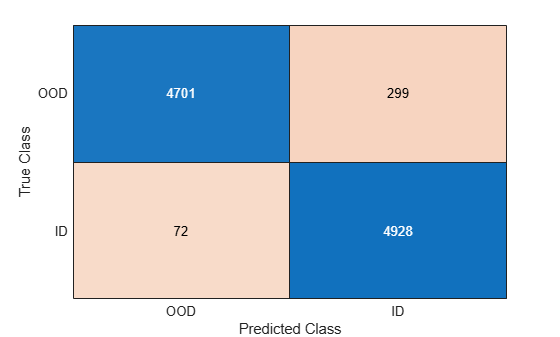Use the `distributionScores` function to find the distribution scores for the ID and OOD data.

```scoresID = distributionScores(discriminator,XID); scoresOOD = distributionScores(discriminator,XOOD); scores = [scoresID',scoresOOD'];```

Use `rocmetrics` to plot a ROC curve to show how well the model is at separating the data into ID and OOD.

```rocObj = rocmetrics(trueDataLabels,scores,"In-Distribution"); plot(rocObj)```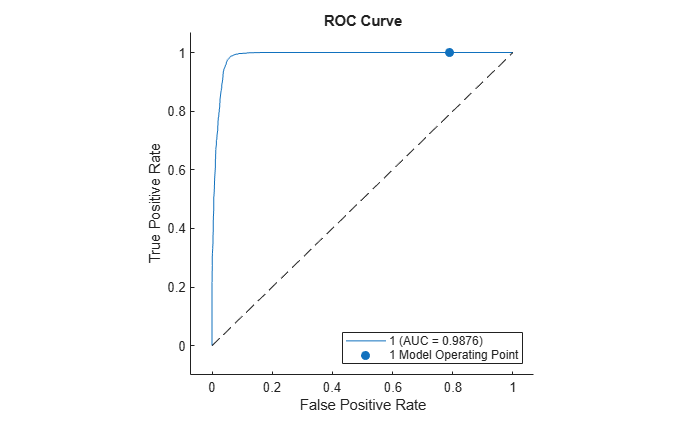## Input Arguments

collapse all

Neural network, specified as a `dlnetwork` object.

In-distribution (ID) data, specified as a formatted `dlarray` object or `[]`. For more information about `dlarray` formats, see the `fmt` input argument of `dlarray`.

At least one of the `XID` and `XOOD` input arguments must be nonempty.

Out-of-distribution (OOD) data, specified as a formatted `dlarray` object or `[]`. For more information about `dlarray` formats, see the `fmt` input argument of `dlarray`.

At least one of the `XID` and `XOOD` input arguments must be nonempty.

Method for computing the distribution confidence scores, specified as one of these values:

Note

Specifying `method` as `"baseline"`, `"odin"`, or `"energy"` is valid only for single-output networks with a softmax layer as the final layer. For example, specifying `net` as a single-output classification network. You can specify `method` as `"hbos"` for any network.

Data Types: `char` | `string`

### Name-Value Arguments

Specify optional pairs of arguments as `Name1=Value1,...,NameN=ValueN`, where `Name` is the argument name and `Value` is the corresponding value. Name-value arguments must appear after other arguments, but the order of the pairs does not matter.

Before R2021a, use commas to separate each name and value, and enclose `Name` in quotes.

Example: `TruePositiveGoal=0.99,Temperature=10`

True positive goal, specified as a scalar in the range [0, 1]. The software returns the threshold that correctly classifies at least this proportion of the ID data as ID.

#### Dependency

If you specify a true positive goal, then `XID` must be nonempty and you cannot specify `FalsePositiveGoal`. If you specify a nonempty `XOOD` value, then the software uses only `XID` to compute the threshold.

If you do not specify the `TruePositiveGoal` and `FalsePositiveGoal` name-value arguments and provide nonempty `XID` and `XOOD` values, then the function does not use the default values and instead optimizes over the true positive rate and false positive rate to find the optimal threshold. For more information, see Algorithms.

Data Types: `single` | `double` | `int8` | `int16` | `int32` | `int64` | `uint8` | `uint16` | `uint32` | `uint64`

False positive goal, specified as a scalar in the range [0, 1]. The software returns the threshold that incorrectly classifies at most this proportion of the OOD data as ID.

#### Dependency

If you specify a false positive goal, then `XOOD` must be nonempty and you must not specify `TruePositiveGoal`. If you specify a nonempty `XID` value, then the software uses only `XOOD` to compute the threshold.

If you do not specify the `TruePositiveGoal` and `FalsePositiveGoal` name-value arguments and provide nonempty `XID` and `XOOD` values, then the function does not use the default values and instead optimizes over the true positive rate and false positive rate to find the optimal threshold. For more information, see Algorithms.

Data Types: `single` | `double` | `int8` | `int16` | `int32` | `int64` | `uint8` | `uint16` | `uint32` | `uint64`

Temperature scaling for the ODIN and energy methods, specified as a positive scalar. The temperature controls the scaling of the softmax scores when the function computes the distribution confidence scores. For more information, see Distribution Confidence Scores.

#### Dependency

To enable this input, specify `method` as `"odin"` or `"energy"`.

Data Types: `single` | `double` | `int8` | `int16` | `int32` | `int64` | `uint8` | `uint16` | `uint32` | `uint64`

Layer names for the HBOS method, specified as a string scalar, character vector, string array, or cell array of character vectors. The default value is the first layer specified in the `OutputNames` property of `net`.

The software computes the distribution confidence scores using the principal components from all of the specified layers. For more information, see Density-Based Methods.

Note

To compute the histograms, the software must first compute the principal component features for each layer. Computing the principal components can be slow for layers with a large output size and can cause you to run out of memory. If you run out of memory, try specifying layers with a smaller output size. For more information, see Density-Based Methods.

#### Dependency

To enable this input, specify `method` as `"hbos"`.

Data Types: `char` | `string` | `cell`

Variance cutoff for the HBOS method, specified as a scalar in the range [0, 1].

The variance cutoff controls the number of principal component features that the software uses to compute the distribution confidence scores. Using a greater number of features takes more computation time. The closer the `VarianceCutoff` value is to `1`, the fewer principal components the software uses to compute the scores. For more information, see Density-Based Methods.

#### Dependency

To enable this input, specify `method` as `"hbos"`.

Data Types: `single` | `double` | `int8` | `int16` | `int32` | `int64` | `uint8` | `uint16` | `uint32` | `uint64`

## Output Arguments

collapse all

Distribution discriminator, returned as a `BaselineDistributionDiscriminator`, `ODINDistributionDiscriminator`, `EnergyDistributionDiscriminator`, or `HBOSDistributionDiscriminator` object.

The function returns this output as a `BaselineDistributionDiscriminator`, `ODINDistributionDiscriminator`, `EnergyDistributionDiscriminator`, or `HBOSDistributionDiscriminator` object when you specify `method` as `"baseline"`, `"odin"`, `"energy"`, or `"hbos"`, respectively.

The `Threshold` property of `discriminator` contains the threshold for separating the ID and OOD data. To access the threshold value, call `discriminator.Threshold`.

collapse all

### In-Distribution and Out-of-Distribution Data

In-distribution (ID) data refers to any data that you use to construct and train your model. Additionally, any data that is sufficiently similar to the training data is also said to be ID.

Out-of-distribution (OOD) data refers to data that is sufficiently different to the training data. For example, data collected in a different way, at a different time, under different conditions, or for a different task than the data on which the model was originally trained. Models can receive OOD data when you deploy them in an environment other than the one in which you train them. For example, suppose you train a model on clear X-ray images but then deploy the model on images taken with a lower-quality camera.

OOD data detection is important for assigning confidence to the predictions of a network. For more information, see OOD Data Detection.

### OOD Data Detection

OOD data detection is a technique for assessing whether the inputs to a network are OOD. For methods that you apply after training, you can construct a discriminator which acts as an additional output of the trained network that classifies an observation as ID or OOD.

The discriminator works by finding a distribution confidence score for an input. You can then specify a threshold. If the score is less than or equal to that threshold, then the input is OOD. Two groups of metrics for computing distribution confidence scores are softmax-based and density-based methods. Softmax-based methods use the softmax layer to compute the scores. Density-based methods use the outputs of layers that you specify to compute the scores. For more information about how to compute distribution confidence scores, see Distribution Confidence Scores.

These images show how a discriminator acts as an additional output of a trained neural network.

Example Data Discriminators

Example of Softmax-Based DiscriminatorExample of Density-Based Discriminator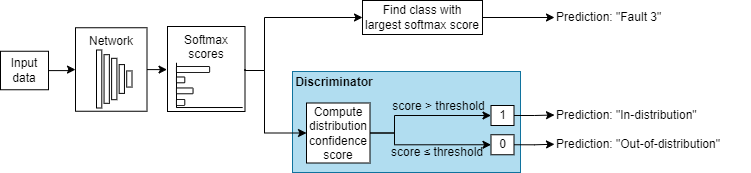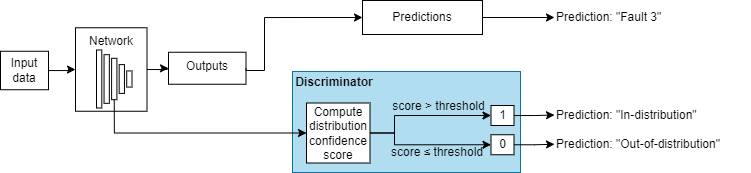### Distribution Confidence Scores

Distribution confidence scores are metrics for classifying data as ID or OOD. If an input has a score less than or equal to a threshold value, then you can classify that input as OOD. You can use different techniques for finding the distribution confidence scores.

Softmax-Based Methods

ID data usually corresponds to a higher softmax output than OOD data. Therefore, a method of defining distribution confidence scores is as a function of the softmax scores. These methods are called softmax-based methods. These methods only work for classification networks with a single softmax output.

Let ai(X) be the input to the softmax layer for class i. The output of the softmax layer for class i is given by this equation:

`${P}_{i}\left(X;T\right)=\frac{{e}^{{a}_{i}\left(X\right)/T}}{\sum _{j=1}^{C}{e}^{{a}_{j}\left(X\right)/T}},$`

where C is the number of classes and T is a temperature scaling. When the network predicts the class label of X, the temperature T is set to `1`.

The baseline, ODIN, and energy methods each define distribution confidence scores as functions of the softmax input.

• The baseline method  uses the maximum of the unscaled output values of the softmax scores:

`$\text{confidence}\left(X\right)=\underset{i}{\mathrm{max}}{P}_{i}\left(X;1\right).$`

• The out-of-distribution detector for neural networks (ODIN) method  uses the maximum of the scaled output of the softmax scores:

• The energy method  uses the scaled denominator of the softmax output:

Density-Based Methods

Density-based methods compute the distribution scores by describing the underlying features learned by the network as probabilistic models. Observations falling into areas of low density correspond to OOD observations.

To model the distributions of the features, you can describe the density function for each feature using a histogram. This technique is based on the histogram-based outlier score (HBOS) method . This method uses a data set of ID data, such as training data, to construct histograms representing the density distributions of the ID features. This method has three stages:

1. Find the principal component features for which to compute the distribution confidence scores:

1. For each specified layer, find the activations using the n data set observations. Flatten the activations across all dimensions except the batch dimension.

2. Compute the principal components of the flattened activations matrix. Normalize the eigenvalues such that the largest eigenvalue is 1 and corresponds to the principal component that carries the greatest variance through the layer. Denote the matrix of principal components for layer l by Q(l).

The principal components are linear combinations of the activations and represent the features that the software uses to compute the distribution scores. To compute the score, the software uses only the principal components whose eigenvalues are greater than the variance cutoff value σ.

Note

The HBOS algorithm assumes that the features are statistically independent. The principal component features are pairwise linearly independent but they can have nonlinear dependencies. To investigate feature dependencies, you can use functions such as `corr` (Statistics and Machine Learning Toolbox). For an example showing how to investigate feature dependence, see Out-of-Distribution Data Discriminator for YOLO v4 Object Detector. If the features are not statistically independent, then the algorithm can return poor results. Using multiple layers to compute the distribution scores can increase the number of statistically dependent features.

2. For each of the principal component features with an eigenvalue greater than σ, construct a histogram. For each histogram:

1. Dynamically adjusts the width of the bins to create $\sqrt{n}$ bins of approximately equal area.

2. Normalize the bins heights such that the largest height is 1.

3. Find the distribution score for an observation by summing the logarithmic height of the bin containing the observation for each of the feature histograms, over each of the layers.

Let f(l)(X) denote the output of layer l for input X. Use the principal components to project the output into a lower dimensional feature space using this equation: ${\stackrel{^}{f}}^{\left(l\right)}\left(X\right)={\left({Q}^{\left(l\right)}\right)}^{T}{f}^{\left(l\right)}\left(X\right)$.

Compute the confidence score using this equation:

where N(l)(σ) is the number of number of principal component with an eigenvalue less than σ and L is the number of layers. A larger score corresponds to an observation that lies in the areas of higher density. If the observation lies outside of the range of any of the histograms, then the bin height for those histograms is 0 and the confidence score is `-Inf`.

Note

The distribution scores depend on the properties of the data set used to construct the histograms .

## Algorithms

The function creates a discriminator using the trained network. The discriminator behaves as an additional output of the network and classifies an observation as ID or OOD using a threshold. For more information, see OOD Data Detection.

To compute the distribution threshold, the function first computes the distribution confidence scores using the method that you specify in the `method` input argument. For more information, see Distribution Confidence Scores. The software then finds the threshold that best separates the scores of the ID and OOD data. To find the threshold, the software optimizes over these values:

• True positive goal — Number of ID observations that the discriminator correctly classifies as ID. To optimize for this value, the ID data `XID` must be nonempty and you must specify `TruePositiveGoal`. If you specify `TruePositiveGoal` as `p`, then the software finds the threshold above which the proportion of ID confidence scores is `p`. This process is equivalent to finding the 100(1-`p`)-th percentile for the ID confidence scores.

• False positive goal — Number of OOD observations that the discriminator incorrectly classifies as ID. To optimize for this value, the OOD data `XOOD` must be nonempty and you must specify `FalsePositiveGoal`. If you specify `FalsePositiveGoal` as `p`, then the software finds the threshold above which the proportion of OOD confidence scores is `p`. This process is equivalent to finding the 100`p`-th percentile for the OOD confidence scores.

If you provide ID and OOD data and do not specify `TruePositiveGoal` or `FalsePositiveGoal`, then the software finds the threshold that maximizes the balanced accuracy $\frac{1}{2}\left(TPR+\left(1-FPR\right)\right)$. If you provide only ID data, then the software optimizes using only `TruePositiveGoal`, whose default is `0.95`. If you provide only OOD data, then the software optimizes using only `FalsePositiveGoal`, whose default is `0.05`.

This figure illustrates the different thresholds that the software chooses if you optimize over both the true positive rate and false positive rate, just the true positive rate, or just the false positive rate.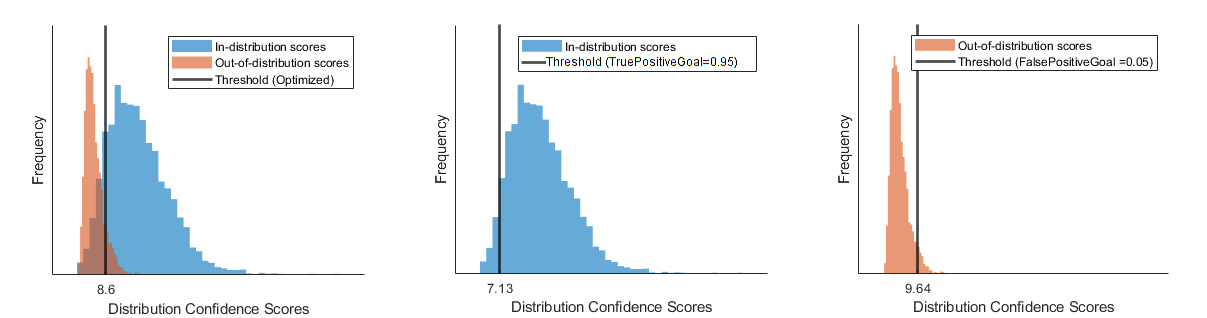Shalev, Gal, Gabi Shalev, and Joseph Keshet. “A Baseline for Detecting Out-of-Distribution Examples in Image Captioning.” In Proceedings of the 30th ACM International Conference on Multimedia, 4175–84. Lisboa Portugal: ACM, 2022. https://doi.org/10.1145/3503161.3548340.

 Shiyu Liang, Yixuan Li, and R. Srikant, “Enhancing The Reliability of Out-of-distribution Image Detection in Neural Networks” arXiv:1706.02690 [cs.LG], August 30, 2020, http://arxiv.org/abs/1706.02690.

 Weitang Liu, Xiaoyun Wang, John D. Owens, and Yixuan Li, “Energy-based Out-of-distribution Detection” arXiv:2010.03759 [cs.LG], April 26, 2021, http://arxiv.org/abs/2010.03759.

 Markus Goldstein and Andreas Dengel. "Histogram-based outlier score (hbos): A fast unsupervised anomaly detection algorithm." KI-2012: poster and demo track 9 (2012).

 Jingkang Yang, Kaiyang Zhou, Yixuan Li, and Ziwei Liu, “Generalized Out-of-Distribution Detection: A Survey” August 3, 2022, http://arxiv.org/abs/2110.11334.

 Lee, Kimin, Kibok Lee, Honglak Lee, and Jinwoo Shin. “A Simple Unified Framework for Detecting Out-of-Distribution Samples and Adversarial Attacks.” arXiv, October 27, 2018. http://arxiv.org/abs/1807.03888.